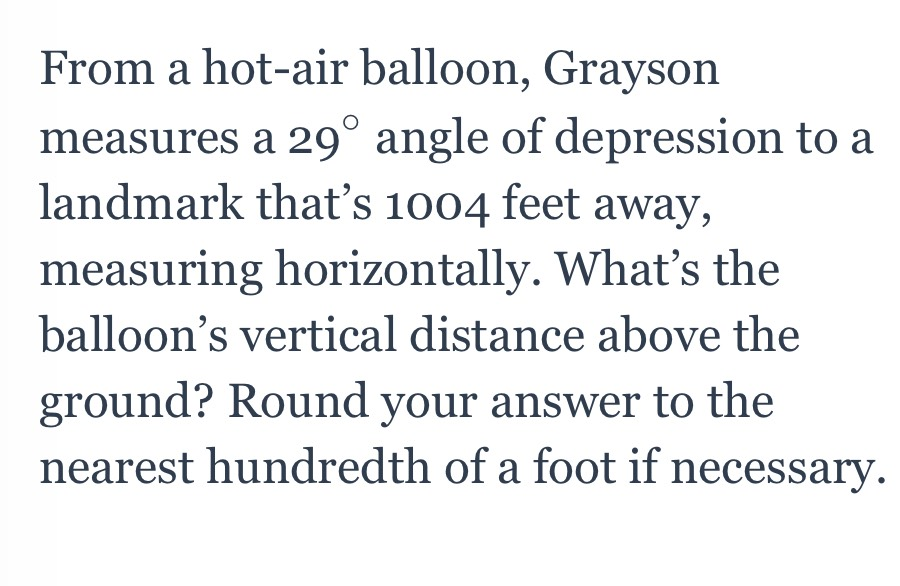### Still have math questions?

Trigonometry
QuestionFrom a hot-air balloon, Grayson measures a $$29 ^ { \circ }$$ angle of depression to a landmark that's $$1004$$ feet away, measuring horizontally. What's the balloon's vertical distance above the ground? Round your answer to the nearest hundredth of a foot if necessary.